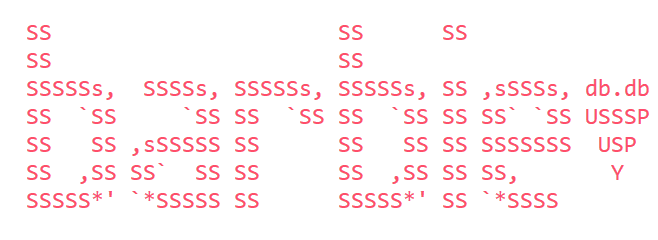Articles 16
Tags 19# PROLOG - Part 8

There is logic behind everything and every rule. We sometimes negate this but every thing and every happening has a logic and reason behind it.

In my last post I wrote about cut, fail and repeat. This time I am going to expand the understanding on internals and data structures.

Terms can be constants, variables or a structure.

• A constant may be an atom or a number - which can be an integer or real number. We defined atom some posts ago. :)
• A variable is a string of letters, digits and / or underscores starting with a upper-case letter or an underscore.
• A structure represents an atomic preposition of predicates. Structures have the form `blub(t1,t2,t3,...,tn)` where blub is our atom and t1,t2,…,tn are our parameters. n is also the arity of our term.

Keep in mind: there is no type declaration in PROLOG. The interpreter will figure it out for you. :)

### Lists

The lists are build of the structure .(H,T) and the atom []. For example, the list `[a,b,c]` is represented by the term `.(a,.(b,.(c,[])))`. A built-in list notation is also available, which defines the head and the tail of the list, as [H,T]. For example, our `[a,b,c] = .(a,.(b,.(c,[]))) = [a|[b,c]] = [a,b|[c]] = [a,b,c|[]] = [a,b,c]`

### Strings

A String is a list of characters’ ASCII codes: `"PROLOG" = [80,82,79,76,79,71]` or `"barbie" = [98, 97, 114, 98, 105, 101].`

Important: Strings are always declared with double quotes. ‘PROLOG’ != “PROLOG” :)

## Run time =)

No, you don’t need to run! But how a program actually works in PROLOG? How can PROLOG solve ALL OUR PROBLEMS (Ok, maybe exaggerating a bit, but let’s say 95% of our problems!). If you look a PROLOG code, it is nothing more than a set of Horn clauses. All facts, rules and questions are stated in a `.pl` file and that’s it! The magic word here is inference.

### Inference

Let’s assume we want to prove `? G1, G2, ..., Gn`. PROLOG is going to check clause per clause from the beginning to the end of the file until it finds a rule which the head unifies with out first goal `G1`. This unification will substitute (the generic unifier) g.

We have three possibilities:

• `C :- P1, ..., Pm` is the found rule. g is so that `C g = G1 g.` is true. Now PROLOG will continue with `? P1g, P2g,...,Pmg, G2g, ..., Gng`.
• `F` is found. g is so that `F g = G1 g` and PROLOG will continue with `? G2g, ..., Gng`.
• Nothing is found. PROLOG returns false.

The proving algorithm terminates, when there is nothing more to prove - the goal is empty - or the prove fails. The interpreter answers the initial question instantiating the substitutions for the variables in the question itself.

The proving algorithm runs top down, depth first and makes use of backtracking.

### Operators

It is possible to declare functions like prefix, posfix or infix operators, connecting it to a precedence. It follows the pattern:

`:- op(precedence, type, name)`

### Unification operator

The infix operator `=` is responsible for establishing the unification relation between two operators.

• `T1=T2` is true if, and only if, a substitution g exists, which when applied to both terms T1 and T2, both are literally equal, this means `T1g==T2g`.
• Two functions unify only if the main atoms of the two terms are equal and have the same arity, and the arguments unify themselves respectively.
• A variable unifies with any term and generates the respectively an instance.
• Two atoms unify if, and only if, the are exactly the same.

### Occurs-Check

Swi-Prolog and Sistus allow the unification of a variable with a term where this variable occurs. That means, it is possible to create recursion or cycles. Formally, this is something not desirable but the occurs-check is performance expensive. In swi-Prolog it is standard on and in Sistus is standard off.

Example of output:

``````% Without occurs-check
?- X = f(X).
X = f(f(f(f(f(f(f(f(f(f(...)))))))))) ?

% With occurs-check
?- X = f(X).
X = f(X).``````

I am pretty sure, this is an huge amount of information to process! Next blog post will be more hands on: Debugging!

by Thaís aka barbie